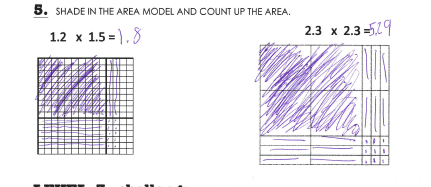# Decimal Multiplication – a Visual Approach

Our 8th grade teachers tell us that of the most confusing ideas for students is the difference between linear units (the sides of a rectangle) and quadratic units (the area of a rectangle). Students routinely confuse x,  x-squared, and x-cubed, without realizing what each represents.

This concept SHOULD reach back to a conceptual development in 4th and 5th grade: the idea of area versus perimeter units.

Our solution: We use spaghetti to illustrate the linear units on the sides of a rectangle. (Actually, if available, linguine works better because it’s flatter!)

Activity:
1. We pass out 2 or 3 spaghetti pieces per student, and papers with different multiplication problems written on the top (a different one for each student). Each problem has factors between 1.1 and 1.5, and has a grid beneath it:The grid has bold marking around a 10 x 10 block.

We have the students break off a spaghetti piece as long as one side of the 10×10 grid. This is now ONE unit long. ONE SPAGHETTI UNIT, not one square unit, obviously. Then we ask them to break off another piece that is about 1/10 as long as the first. They glue these down on the left and top edges of the rectangle assigned on their sheet.

These are the SIDES of the rectangle. This is when all our work with blocks and area models through 4th and 5th grade pays off!

We have the students color in the WHOLE, the TENTHS and the HUNDREDTHS.

They are familiar with the appearance of tenths and hundredths from our games with decimal cards (see last post). They can count up the total value of the product; the area of the rectangle created.

From this point on, all our teachers can use the key word “Spaghetti Units” when they are referring to perimeter, length, or linear units in algebra. It gives us a common visual language that means something to students.

Follow up:  It is important to keep this at the visual level for a couple weeks. This conceptual introduction to decimal multiplication builds number sense and estimation skills. In a week or two, we will move on to multiplication with decimals LESS than one. Here’s the  word doc for these grids.Here are some examples of student work:Whenever students struggle with this (for example, seeing the SIX tenths on the 2 sides of model of 2.3 x 2.3 above) we simply ask them to lay out the BLOCKS for that problem, and it clears up. The concept is always best developed at the concrete level.